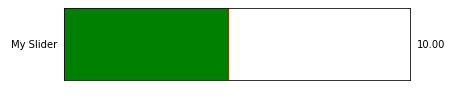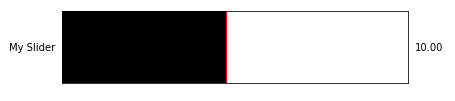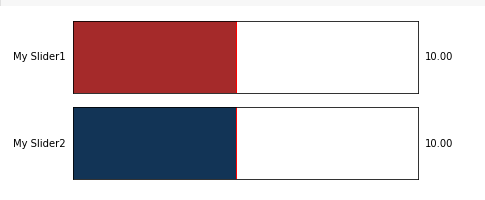# Matplotlib – Change Slider Color

• Last Updated : 08 Oct, 2021

In this article, we will see how to change the slider color of a plot in Matplotlib. First of all, let’s learn what is a slider widget. The Slider widget in matplotlib is used to create a scrolling slider, and we can use the value of the scrolled slider to make changes in our python program. By default, the color of the slider is Blue so, we are going to learn to change the color of the slider.

Syntax: Slider(dimensions, name, minimumValue, maximumValue, initialValue, color);

Parameters:

• dimensions: This parameter takes plt.axes() object to determine dimensions of slider
• name: Name of slider
• minimumValue: Minimum possible value of slider
• maximumValue: Maximum possible value of slider
• initialValue: Initial value of slider
• color: color of slider fill

### Installation

Windows, Linux, and macOS distributions have matplotlib and most of its dependencies as wheel packages. Run the following command to install matplotlib package :

`python -mpip install -U matplotlib`

Note: Slider has a lot of arguments. We are interested in the color argument.

Example 1:

Using the color name, we modify the color of the slider. There are ‘red’, ‘blue’, ‘green’, ‘yellow’, ‘brown’, etc… color names available.

## Python

 `# import libraries``import` `matplotlib.pyplot as plt``from` `matplotlib.widgets ``import` `Slider` `# define dimensions``width ``=` `0.8``height ``=` `0.25``minValue ``=` `1``maxValue ``=` `20` `# Create dimensions of slider``dimentions_of_slider ``=` `plt.axes([``0``, ``0``, width, height])` `# Create slider``mySlider ``=` `Slider(dimentions_of_slider, ``'My Slider'``,``                  ``minValue, maxValue, valinit``=``10``,``                  ``color``=``'green'``)` `# Show Graph``plt.show()`

Output:Example 2:

This example is similar to the above example, but we are using hex code for defining the color. When we need the exact color we want we use HEX Code of color.

## Python

 `# import libraries``import` `matplotlib.pyplot as plt``from` `matplotlib.widgets ``import` `Slider` `# define dimensions``width ``=` `0.8``height ``=` `0.25``minValue ``=` `1``maxValue ``=` `20` `# Create dimensions of slider``dimentions_of_slider ``=` `plt.axes([``0``, ``0``, width, height])` `# Create slider``# Notice the HEX color code below``mySlider ``=` `Slider(dimentions_of_slider, ``'My Slider'``,``                  ``minValue, maxValue, valinit``=``10``,``                  ``color``=``'#000000'``)` `# Show Graph``plt.show()`

Output:Example 3:

In this example, we are creating multiple sliders with different colors using different formats to mention the colors.

## Python

 `# import libraries``import` `matplotlib.pyplot as plt``from` `matplotlib.widgets ``import` `Slider` `# define dimensions``width ``=` `0.8``height ``=` `0.25``minValue ``=` `1``maxValue ``=` `20` `# define sliders``dimentions_of_slider1 ``=` `plt.axes([``0``, ``0.3``, width, height])``dimentions_of_slider2 ``=` `plt.axes([``0``, ``0``, width, height])` `# Using name of Color``mySlider1 ``=` `Slider(dimentions_of_slider1, ``'My Slider1'``,``                   ``minValue, maxValue, valinit``=``10``,``                   ``color``=``'brown'``)` `# Using HEX Code of Color``mySlider2 ``=` `Slider(dimentions_of_slider2, ``'My Slider2'``,``                   ``minValue, maxValue, valinit``=``10``,``                   ``color``=``'#123456'``)` `# show the plot``plt.show()`

Output:My Personal Notes arrow_drop_up